KING FAHD UNIVERSITY OF PETROLUEM AND MINERALS

Faculty of Sciences   Prep Year math program

Math 001 - Term 031

Question1

Given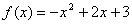.

a) Find the vertex

b) Find the equation of the axis of symmetry .

c) Find the range of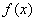d) Sketch the graph of. ( Show the axis of symmetry ,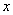-intercept(s),and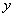-intercept(s) ).

e) Determine the interval in whichis increasing .

f) Determine the interval in whichis decreasing .

Question2

Identify if the function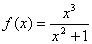is an even  function, an odd function,

Question3

Study the symmetry (about-axis ,-axis , and the origin) for the equation :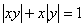..

Question4

Use the  graph of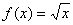to sketch the graph of :                                   (3 marks)1)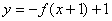2)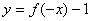Question5

Find the difference quotient of

1)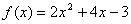.

2)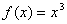Question6

i)                    Let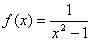and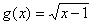.Find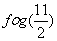ii)                   Let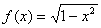find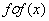Question7

If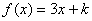and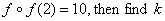.

Question8

Let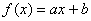where a, b are constants. If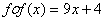, find a and b.